Problem

# A 1-gal tank initially is empty, and we want to fill it with 0.03 lbm R-410a. The R-410a c...

A 1-gal tank initially is empty, and we want to fill it with 0.03 lbm R-410a. The R-410a comes from a line with saturated vapor at 20 F. To achieve the desired amount, we cool the tank while we fill it slowly, keeping the tank and its content at 20 F. Find the final pressure to reach before closing the valve and the heat transfer.

#### Step-by-Step Solution

Solution 1

Given

Volume of the tank,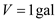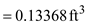Mass of the R-410a,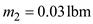Inlet temperature,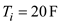Final temperature,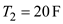From the continuity equation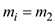From the energy equation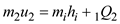At state2

Specific volume,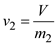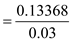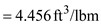For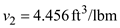andFrom super heated R-410a tables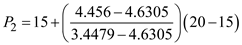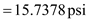Final pressure,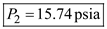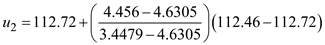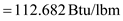From saturated R-410a tables

At state i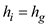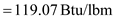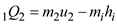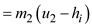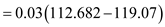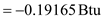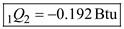Solution 2

Given

Volume of the tank, $$V=1$$ gal

$$=0.13368 \mathrm{ft}^{3}$$

Mass of the $$\mathrm{R}-410 \mathrm{a}, m_{2}=0.03 \mathrm{lbm}$$

Inlet temperature, $$T_{i}=20 \mathrm{~F}$$

Final temperature, $$T_{2}=20 \mathrm{~F}$$

From the continuity equation

$$m_{i}=m_{2}$$

From the energy equation

$$m_{2} u_{2}=m_{i} h_{i}+{ }_{1} Q_{2}$$

At state2

Specific volume, $$v_{2}=\frac{V}{m_{2}}$$

$$=\frac{0.13368}{0.03}$$

$$=4.456 \mathrm{ft}^{3} / \mathrm{lbm}$$

For $$v_{2}=4.456 \mathrm{ft}^{3} / \mathrm{lbm}$$ and $$T_{2}=20 \mathrm{~F}$$

From super heated R-410a tables

$$P_{2}=15+\left(\frac{4.456-4.6305}{3.4479-4.6305}\right)(20-15)$$

$$=15.7378 \mathrm{psi}$$

Final pressure, $$P_{2}=15.74$$ psia

$$u_{2}=112.72+\left(\frac{4.456-4.6305}{3.4479-4.6305}\right)(112.46-112.72)$$

$$=112.682 \mathrm{Btu} / \mathrm{lbm}$$

From saturated R-410a tables

At state i

$$h_{i}=h_{g}$$

$$=119.07 \mathrm{Btu} / \mathrm{lbm}$$

$${ }_{1} Q_{2}=m_{2} u_{2}-m_{i} h_{i}$$

$$=m_{2}\left(u_{2}-h_{i}\right)$$

$$=0.03(112.682-119.07)$$

$$=-0.19165 \mathrm{Btu}$$

$${ }_{1} Q_{2}=-0.192 \mathrm{Btu}$$

Add your Solution
Textbook Solutions and Answers Search
Solutions For Problems in Chapter 6